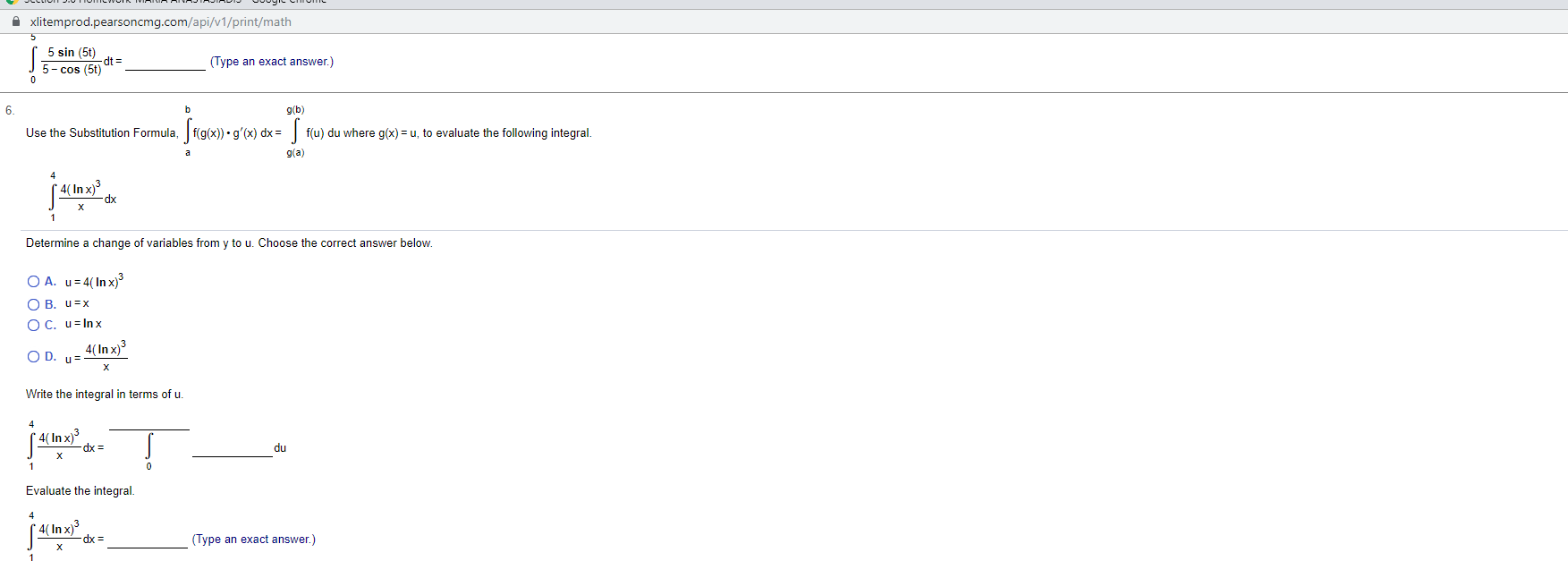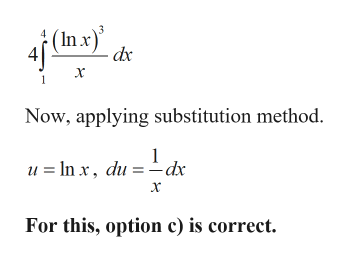# xlitemprod.pearsoncmg.com/api/v1/print/math5 sin (5t)dt =5 cos (5t)(Type an exact answer.)06g(b)Use the Substitution Formula,f(g(x)). g'(x) dxf(u) du where g(x)= u, to evaluate the following integralg(a)(4(Indk1Determine a change of variables from y to u. Choose the correct answer below.O A. u 4(In x)3O B. u xO C. u Inx4(In x)3O D. uXWrite the integral in terms of u.4(In x)3du= xp0Evaluate the integral4( In x)sdx(Type an exact answer.)

Question
21 views

Can you help me step by step pleasehelp_outlineImage Transcriptionclosexlitemprod.pearsoncmg.com/api/v1/print/math 5 sin (5t) dt = 5 cos (5t) (Type an exact answer.) 0 6 g(b) Use the Substitution Formula,f(g(x)). g'(x) dx f(u) du where g(x)= u, to evaluate the following integral g(a) (4(Indk 1 Determine a change of variables from y to u. Choose the correct answer below. O A. u 4(In x)3 O B. u x O C. u Inx 4(In x)3 O D. u X Write the integral in terms of u. 4(In x)3 du = xp 0 Evaluate the integral 4( In x)s dx (Type an exact answer.) fullscreen
check_circle

Step 1

Given:

Step 2

Calculation:

First, we have to take the constant out from the integral.help_outlineImage Transcriptionclose(In.x) - dx Now, applying substitution method. u =In x, du -dx х For this, option c) is correct. fullscreen
Step 3

### Want to see the full answer?

See Solution

#### Want to see this answer and more?

Solutions are written by subject experts who are available 24/7. Questions are typically answered within 1 hour.*

See Solution
*Response times may vary by subject and question.
Tagged in

### Integration